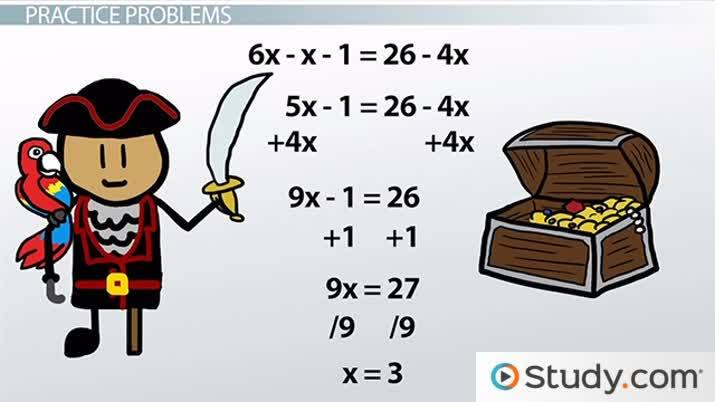# Solving Linear Equations: Practice Problems

Lesson Transcript
Instructor: Jeff Calareso

Jeff teaches high school English, math and other subjects. He has a master's degree in writing and literature.

With practice, linear equations can be straightforward to solve. In this lesson, we'll define linear equations and learn how to solve them. We'll look at multiple practice problems and walk through solving each one.

## X Marks the Spot

X. X is everywhere. It's the checkbox on the user agreement we never read. It marks railroad crossings and superhero uniforms. And, it's at the end of every pirate map. In all these examples, x stands for something. For example, on that pirate map, x is where you'll find the buried treasure. In algebra, x is sort of like a marker for treasure, if by treasure we mean a number. That's because x is a common variable. And, a variable is a symbol used to represent a number.

Here we're going to solve for x in linear equations. It's just like following a pirate map, where we follow the clues until we know where x is. Fortunately, with linear equation solving, you're much less likely to lose a leg or an eye. And, scurvy is very rare. First, let's do a quick review of what these equations are.An error occurred trying to load this video.

Try refreshing the page, or contact customer support.

Coming up next: What is a System of Equations?

### You're on a roll. Keep up the good work!

Replay
Your next lesson will play in 10 seconds
• 0:05 X Marks the Spot
• 0:56 Linear Equations
• 1:42 Solving Linear Equations
• 2:19 Practice Problems
• 5:21 Lesson Summary
Save Save

Want to watch this again later?

Timeline
Autoplay
Autoplay
Speed Speed

## Linear Equations

A linear equation is simply an algebraic expression that represents a line. These equations commonly contain one or two variables, usually x or y. These are called first-degree equations because the variable's exponent is always one.

We won't see anything like x^2 or x^3. Those may get you lines like what you'd actually see on a pirate map. But, our pirate map has straight lines. It's much easier that way. You also won't see things like x times y, x over y or the square root of x. That's for pirates who travel through time and space.

Oh, and the linear equations we'll be solving here only have one variable, not two or more. I mean, if we had both x and y, how do we know which one has the treasure and which one is a trap? Pirates are big into traps.

## Solving Linear Equations

As I mentioned before, to solve one of these equations, we're trying to solve for x. If you have x - 4 = 10, then 10 isn't where the treasure is. It's where x - 4 is. And, that's just not what we need.

To solve this equation, we need to get x alone on one side of the equation. Here, we do that by adding 4 to each side. That gets us x = 14. So, that's our treasure. I know that's a modest haul, but it was a basic equation.

For all of these equations, we'll always just do whatever we can to isolate x. If we have 2x = 6, we divide by 2 to get x = 3. Just keep your focus on x.

## Practice Problems

Now that we know what to do, let's go after some serious treasure. Let's start with x + 2 = 9. This looks like the first one we saw. This is like Pirate Treasure Hunting 101. Let's subtract 2 from both sides to get x = 7. It's another small treasure, but it is ours.

What about 3x - 4 = 11? Let's add 4 to each side. Then divide by 3. We get x = 5. That's a little more gold. We could buy ourselves a parrot.

Here's another: 4x - 9 = 2x - 3. We have xs on both sides, so we need to move them around. Let's subtract 2x from both sides. Now add 9 to both sides. Then divide by 2. That's x = 3. I think we can get a pretty sweet sword with that.

To unlock this lesson you must be a Study.com Member.

### Register to view this lesson

Are you a student or a teacher?